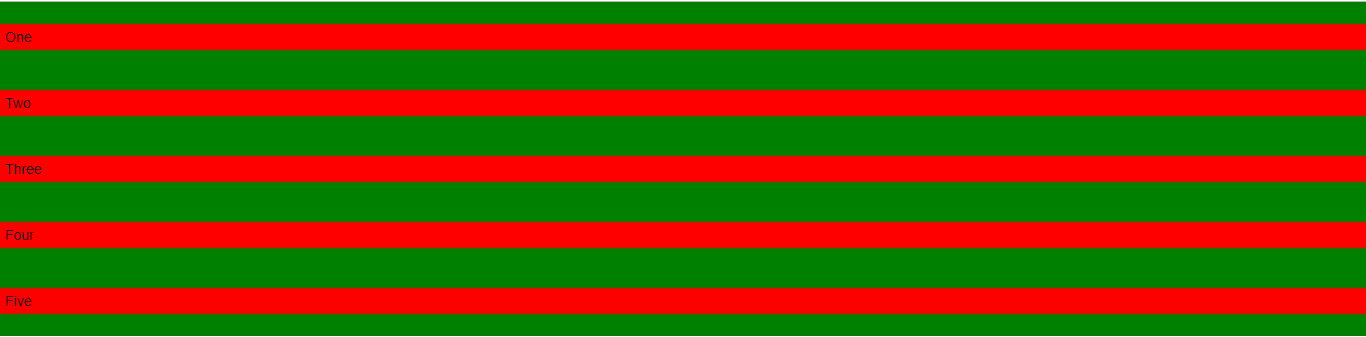Open In App

# How to make flexbox children 100% height of their parent using CSS?

CSS Flexbox is an awesome tool to create website layouts. Making a flex-box child 100% the height of their parent can be done in two ways. It is a little tricky because, certainly it will display an error.

For example, the child may flow out of the parent boundary or it may not get up to 100% height that you will see in your browser output.

Example 1: This example makes a child flex-box of height 100% using CSS

## html

 ```<``html``>``  ` `<``head``>``    ``<``title``>``        ``Make flex-box child height 100%``    ``` `    ``<``style``>``        ``* {``            ``padding: 0;``            ``margin: 0;``        ``}` `        ``.container {``            ``width: 100vw;``            ``height: 50vh;``            ``background-color: green;``            ``display: flex;``            ``justify-content: center;``            ``align-items: center;``        ``}` `        ``.child-div {``            ``height: 100%;``            ``background-color: red;``            ``margin: 0 20px;``        ``}``    ````` `<``body``>``    ``<``div` `class``=``"container"``>``        ``<``div` `class``=``"child-div"``>``            ``One``        ````        ``<``div` `class``=``"child-div"``>``            ``Two``        ````        ``<``div` `class``=``"child-div"``>``            ``Three``        ````        ``<``div` `class``=``"child-div"``>``            ``Four``        ````        ``<``div` `class``=``"child-div"``>``            ``Five``        ````    ``````  ` ``

Output:Example 2: The second way to achieve this is by using the align-items property in the parent div to ‘stretch’. It makes every .child-div 100% height of its parent height.

## html

 ```<``html``>``  ` `<``head``>``    ``<``title``>``        ``Make flex-box child height 100%``    ``` `    ``<``style``>``        ``* {``            ``padding: 0;``            ``margin: 0;``        ``}` `        ``.container {``            ``width: 100vw;``            ``height: 50vh;``            ``background-color: green;``            ``display: flex;``            ``justify-content: center;``        ``}` `        ``.child-div {``            ``background-color: red;``            ``margin: 0 20px;``        ``}``    ````` `<``body``>``    ``<``div` `class``=``"container"``>``        ``<``div` `class``=``"child-div"``>``            ``One``        ````        ``<``div` `class``=``"child-div"``>``            ``Two``        ````        ``<``div` `class``=``"child-div"``>``            ``Three``        ````        ``<``div` `class``=``"child-div"``>``            ``Four``        ````        ``<``div` `class``=``"child-div"``>``            ``Five``        ````    ``````  ` ``

Output:Example 3: This example makes the width of the child 100% of their parent.

## html

 ```<``html``>``  ` `<``head``>``    ``<``title``>``        ``Make flex-box child width 100%``    ``` `    ``<``style``>``        ``* {``            ``padding: 0;``            ``margin: 0;``        ``}` `        ``.container {``            ``width: 100vw;``            ``height: 50vh;``            ``background-color: green;``            ``display: flex;``            ``flex-direction: column;``            ``justify-content: center;``        ``}` `        ``.child-div {``            ``background-color: red;``            ``margin: 20px 0;``            ``padding: 5px;``        ``}``    ````` `<``body``>``    ``<``div` `class``=``"container"``>``        ``<``div` `class``=``"child-div"``>``            ``One``        ````        ``<``div` `class``=``"child-div"``>``            ``Two``        ````        ``<``div` `class``=``"child-div"``>``            ``Three``        ````        ``<``div` `class``=``"child-div"``>``            ``Four``        ````        ``<``div` `class``=``"child-div"``>``            ``Five``        ````    ``````  ` ``

Output:Supported Browser: